• 基于KMeans聚类的协同过滤推荐算法可运用于基于...KMeans聚类算法聚类算法中最基础最常用、最重要的聚类算法。KMeans聚类算法首先需要确定N个初始中心点，初始中心点的选择对聚类结果影响很大，常用的初始中心点的...
基于KMeans聚类的协同过滤推荐算法可运用于基于用户和基于项目的协同过滤推荐算法中，作为降低数据稀疏度和提高推荐准确率的方法之一，一个协同过滤推荐过程可实现多次KMeans聚类。
一、基于KMeans聚类的协同过滤推荐算法推荐原理
KMeans聚类算法是聚类算法中最基础最常用、最重要的聚类算法。KMeans聚类算法首先需要确定N个初始中心点，初始中心点的选择对聚类结果影响很大，常用的初始中心点的选择有随机选择、自定义、采用Canopy聚类算法结果作为初始中心点，然后是重复遍历点与簇中心的距离，并不断修正簇中心点，可设置遍历次数和点与簇中心的最小距离影响聚类结果。
聚类的数据可以是一维数组、二维数组、N维数组，其中一维和二维数组的聚类结果便于可视化，一维数组初始中心点的选择采用自定义，这样聚类效果偏好，二维和N维数组采用Canopy聚类算法的聚类结果作为初始中心点效果偏好。下面介绍一下Canopy聚类算法：
Canopy聚类算法
Canopy聚类算法的基本原则是：首先应用成本低的近似的距离计算方法高效的将数据分为多个组，这里称为一个 Canopy，暂时翻译为“树冠”，Canopy之间可以有重叠的部分；然后采用严格的距离计算方式准确的计算在同一Canopy中的点，将他们分配与最合适的簇中。Canopy聚类算法经常用于K均值聚类算法的预处理，用来找合适的k值和簇中心。
下面详细介绍一下创建Canopy的过程：初始，假设我们有一组点集S，并且预设了两个距离阈值T1、T2（T1>T2）；然后选择一个点，计算它与S中其他点的距离（这里采用成本很低的计算方法），将距离在T1以内的放入一个Canopy中，同时从S中去掉那些与此点距离在T2以内的点（这里是为了保证和中心距离在T2以内的点不能再作为其他Canopy的中心），重复整个过程直到S为空为止。
二、代码实现
本文主要是java语言开发，数据是1000个随机点，程序分别使用KMeans实现，Canopy算法实现，KMeans+Canopy算法实现。聚类效果如下图：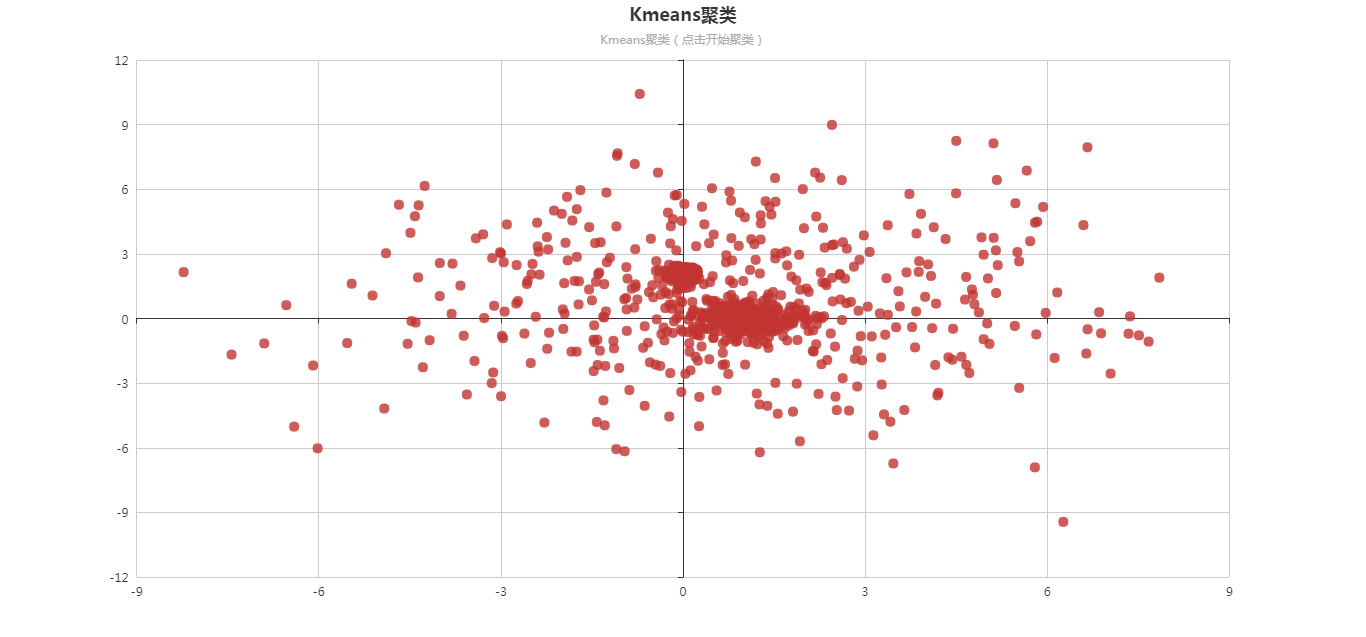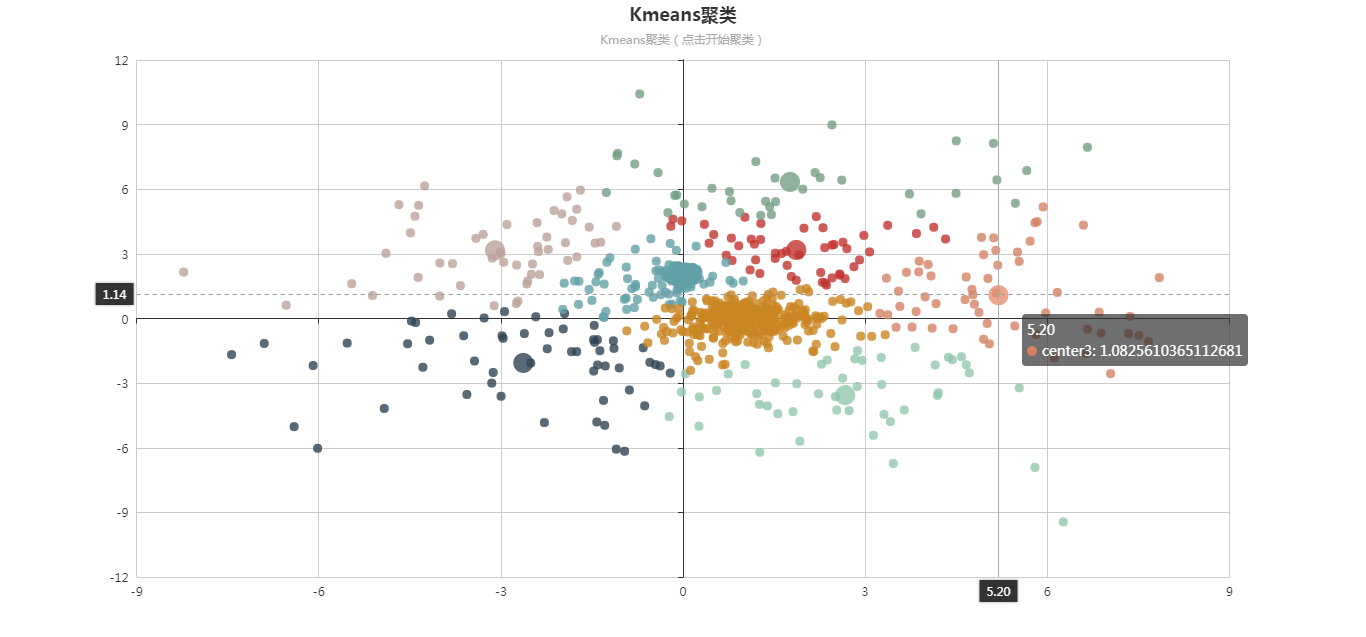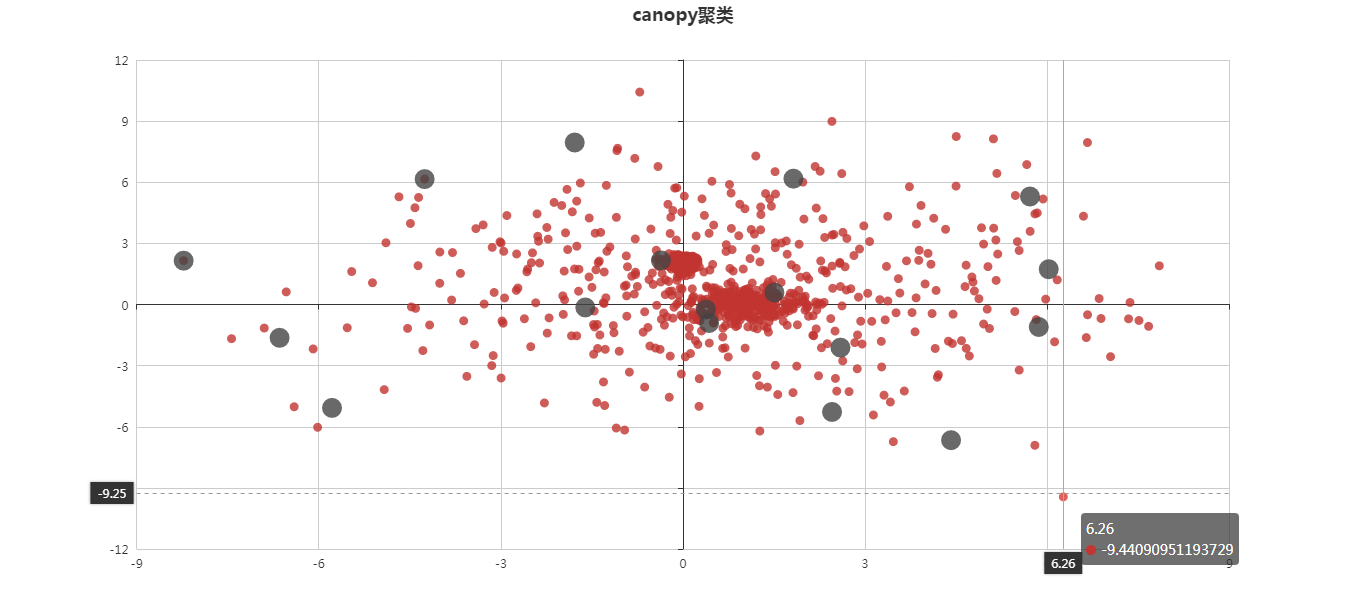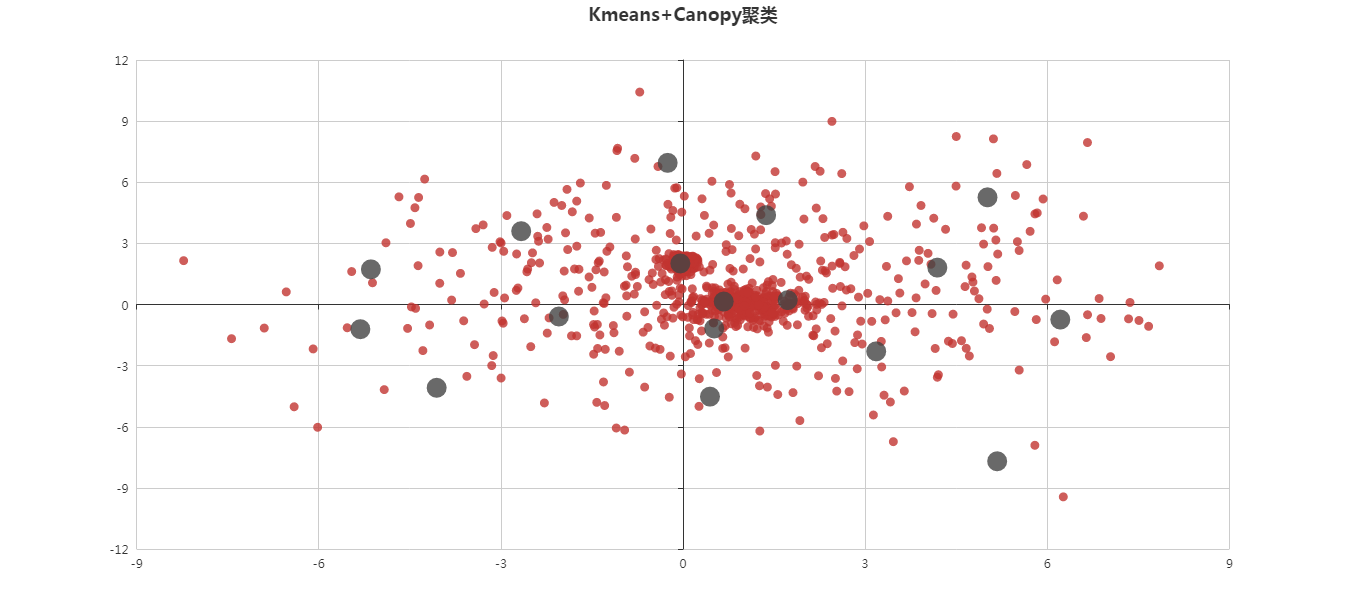该程序仅供学习和测试使用。
作者专业长期研究各种协同过滤推荐算法，欢迎留言、私信互相交流学习，后续会不断更新不同的协同过滤推荐算法，欢迎关注。


展开全文java 深度学习 机器学习
• 用MATLAB实现将K均值聚类算法用于图像的分割
目的
实现将K均值聚类算法用于图像的分割（以下采用一张比较常见的图片,这张图片即为下面使用的test1.png，当然，也可采用别的图片，用小黄人做实验只是因为效果比较OK）k均值聚类结果受到所选聚类中心的个数K和其初始位置以及模式样本的几何性质及读入次序等的影响。实际应用中需要试探不同的K值和选择不同的聚类中心起始值。
道理是这样，但是我们是直接用MATLAB里面的kmeans函数（用	 help(‘kmeans’)	可以直接查看kmeans的相关信息），聚类起始点我们并不需要考虑。代码中我们重点关照一下K，最好把不同的K得出的效果放在同一个figure中，这样对比起来效果比较明显。此外，比较有可能影响结果的应该就是模式样本的几何性质了，也就是图片的前景背景的占比，RGB占比等等。(所以建议多试试几张图看看对比)
代码
close all;
clear all;
clc;

k=2;

figure;
subplot(2,2,1);
imshow(org),title('原始图像');    %显示原图像

% 接下来需要知道图片的尺寸（长和宽），如若直接对RGB图像进行操作，如下
% [m,n,p]=size(org)                     %m,n为所求，p=3为通道数

% 或者用下面的这种方法，转化为灰度图再求
gray=rgb2gray(org);
[m,n]=size(gray);

% 将图像进行RGB——3通道分解
% org(:, :, 1)......分别代表rgb通道
A = reshape(org(:, :, 1), m*n, 1);
B = reshape(org(:, :, 2), m*n, 1);
C = reshape(org(:, :, 3), m*n, 1);
data = [A B C];                              % r g b分量组成样本的特征，每个样本有三个属性值，共width*height个样本

% 第二张图
% kmeans第一个参数: N*P的数据矩阵，N为数据个数，P为单个数据维度
res = kmeans(double(data), k);
result = reshape(res, m, n);             % 反向转化为图片形式
subplot(2,2,2);
% label2rgb功能是转换标记矩阵到RGB图像
imshow(label2rgb(result)),title(strcat('K=',num2str(k),'时RGB通道分割结果'));   % 显示分割结果

% 第三张图
res = kmeans(double(data), k+1);
result = reshape(res, m, n);
subplot(2,2,3);
imshow(label2rgb(result)),title(strcat('K=',num2str(k+1),'时RGB通道分割结果'));

% 第四张图
res = kmeans(double(data), k+2);
result = reshape(res, m, n);
subplot(2,2,4);
imshow(label2rgb(result)),title(strcat('K=',num2str(k+2),'时RGB通道分割结果'));

结果如下如果说非要讨论距离、起始点等因素的话，可以调用kmeans的另一种形式进行实现。如下。具体参数信息依然是用 	help('kmeans')	查看。
[...] = kmeans(...,param1,val1,param2,val2,...)

参数不清楚的可以参考这个链接


展开全文模式识别 matlab 图像分割
• FCM进行图像分割的评价指数使用FCM聚类算法进行图像分割Bezdek划分系数Xie_Beni系数重构错误率V_RE如何改变文本的样式插入链接与图片如何插入一段漂亮的代码片生成一个适合你的列表创建一个表格设定内容居中、居左、...
FCM进行图像分割的评价指数使用FCM聚类算法进行图像分割Bezdek划分系数Xie_Beni系数重构错误率V_REPBM指标代码[^2]参考资料
使用FCM聚类算法进行图像分割
使用无监督算法进行图像分割，往往这类图像没有真实值，没办法用有监督的指标进行评价；这里介绍三种内部评价指标：Bezdek划分系数、Xie_Beni系数、重构错误率V_RE和PBM指标。
Bezdek划分系数
$V_{PC}$ 是Bezdek划分系数，它主要目的是重新刻画分割后的像素的隶属度，定义如下：
$V_{P C}=\frac{1}{n}\sum_{i=1}^{c} \sum_{j=1}^{n} u_{i j}^{2}$
即所有元素隶属于各个分类隶属度的平方和，对图像进行分割时，性能较优的聚类算法是使图像中
像素归属于某一类的隶属度尽可能地大，反之属于其他聚类的隶属度尽可能的小，即$V_{PC}$ 的值越大，表明聚类的效果越好。1
Xie_Beni系数
$V_{XB}$是 Xie-Beni系数，其定义为：
$V_{\mathrm{XB}}=\frac{\sum_{i=1}^{c} \sum_{j=1}^{n} u_{i j}^{2}\left\|x_{j}-v_{i}\right\|^{2}}{n\left(\min _{j \neq k}\left\|v_{j}-v_{k}\right\|^{2}\right)}$
该系数反映了图像聚类内部的一种距离度量，在进行图像分割时，期望聚类内部的像素更加紧密，即 $V_{XB}$ 越小，则说明聚类的效果越好。
重构错误率V_RE
$V_{RE}$是图像的重构错误率，其定义如下：
$V_{RE}=\frac{1}{n} \sum_{i=1}^{n}\left\|I^{\prime}(i)-I(i)\right\|^{2}$
它是分析分割后的重构图像与初始图像间的差距，$I^{\prime}(i)$为重构图像的第 $i$个像素的灰度值，定义为：
$I^{\prime}(i)=\frac{\sum_{k=1}^{c} u_{k i}^{2} I(i)}{\sum_{k=1}^{c} u_{k i}^{2}}$
对图像进行分割后，新的隶属度和聚类中心对图像进行重构，重构后的图像应与原图像尽可能的相似，即表明 $V_{RE}$的值越小，算法的分割效果越好。
PBM指标
PBM指标是一种聚类有效性指标，定义如下：
$P B M =\left(\frac{1}{K} \times \frac{E_{1}}{E_{K}} \times D_{K}\right)^{2}$
$PBM$由三部分组成，分别是$1 / K$ 、$E_{1} / E_{k}$ 和 $D_{K}$。第一项中$K$是提前给定的图像分割类别数，第二项中$E_{1}$对于一个给定的数据集，为一个常值。而$E_{k}$是待分类数据与个体中聚类中心的模糊距离之和，$PBM$值会随着$E_{k}$的减小而增大。第三项中 $D_{K}$是个体中的所有聚类中心对之间的最大距离。$PBM$指标达到最大值，取得最优划分。
代码2

function [Bezdek_V, Xie_Beni_V, V_RE, V_PBM] = Evaluation_index(I, U, V)

% Evaluation_index为聚类分割评价指数
% 输入: 原始待聚类数据I, 隶属度矩阵U, 聚类中心V
% 输出: Bezdek划分系数, Xie_Beni系数, 重构错误率V_RE,V_PBM

%%
% 原始待聚类数据I,n代表样本数目,d代表每个样本的维数
[n,d] = size(I);

% 隶属度矩阵U,c代表聚类类别数目,n代表样本数目
[c,n] = size(U);

% 聚类中心V,c为聚类中心的数目,d代表每个样本的维数
[c,d] = size(V);

%% Bezdek划分系数
% 所有元素隶属于各个分类隶属度的平方和
Bezdek_V = sum(sum(U.^2)) / n ;

%% Xie_Beni系数、重构错误率V_RE
% Xie_Beni系数
Xie_Beni_V = 0;

% 初始化重构图像像素值
I_RE = zeros(n,d);

% XB指数分母项,最近类间距离
% 初始化最近的类间距离的平方为 前两个聚类中心的距离平方
% 欧氏距离：向量按元素求幂，再求和
min_V = sum((V(1,:)-V(2,:)).^2);

for i = 1:c
for j = 1:c
if(i-j ~= 0 && sum((V(i,:)-V(j,:)).^2) < min_V)
% 排除自己和自己之间的距离0之后，遇到更小的类间距离 即 更新初始类间距离
min_V = sum((V(i,:)-V(j,:)).^2);
end
end
end

%%
% 遍历样本
for i = 1:n

% temp表示重构图像像素值的分子,temp1表示其分母
temp = 0;
temp1 = 0;

% 遍历类别
for j = 1:c

% XB指数的分子
Xie_Beni_V = Xie_Beni_V + U(j,i)^2 * sum((I(i,:)- V(j,:)).^2);

% 重构图像像素值的分子和分母
temp = temp + U(j,i)^2*I(i,:);
temp1 = temp1 + U(j,i)^2;
end

% 重构图像像素值
I_RE(i,:) = temp / temp1;
end

% 最终的XB指数等于分子除以分母,n为样本数目,min_V为最小类间距离
Xie_Beni_V = Xie_Beni_V / (n * min_V);

% 重构错误率V_RE
V_RE = sum(sum((I_RE - I).^2)) / n;

%% PBM指标

% 计算最大类间距离
max_V = sqrt(sum((V(1,:)-V(2,:)).^2));

for i = 1:c
for j = 1:c
if(i-j ~= 0 && sqrt(sum((V(i,:)-V(j,:)).^2)) > max_V)
% 排除自己和自己之间的距离0之后，遇到更大的类间距离 即 更新初始类间距离
max_V = sqrt(sum((V(i,:)-V(j,:)).^2));
end
end
end

% 初始化Ek
Ek = 0;

for i = 1:n
for j = 1:c
Ek = Ek + U(j,i) * sqrt(sum((I(i,:)- V(j,:)).^2));
end
end

% 初始化E1
E1 = 0;
for i = 1:n
E1 = E1 + U(1,i) * sqrt(sum((I(i,:)- V(1,:)).^2));
end

% V_PBM
V_PBM = (E1 * max_V / (c * Ek))^2;

end


参考资料

王民, 李媛, 张立材. 基于纹理特征的盲道区域分割算法[J]. 信息通信, 2017(7). ↩︎

FCM算法中评价指数matlab程序. ↩︎


展开全文机器学习
• 聚类算法由于其算法流程简单、计算简洁与 Kmeans 算法相比不容易陷入局部最优解，能够对高维度、非常规分布的数据进行聚类...谱聚类算法是利用图谱理论来进行算法分析，思想是把数据分析问题看成是的最优分割问题。

转自： http://blog.csdn.net/aspirinvagrant/article/details/41700025

谱聚类算法

谱聚类算法由于其算法流程简单、计算简洁与 Kmeans 算法相比不容易陷入局部最优解，能够对高维度、非常规分布的数据进行聚类。谱聚类算法是利用图谱理论来进行算法分析，思想是把数据分析问题看成是图的最优分割问题，将数据样本看成是各个数据点，然后将数据点描绘成一个图表，根据图表关系计算出相应的相似矩阵，找到一种最优分割方法计算出相似矩阵的最小特征向量，最后利用相应算法得出最后的聚类结果。

谱聚类算法是将样本点看成为一个个顶点，将顶点之间用带权的边连接起来，带权的边可以看成是顶点之间的相似度。聚类从而可以看成如何分割这些带权的边，继而将聚类问题转化为怎么进行图分割的问题，但是如果这样的话新的问题又产生了，那就是怎样找到一种最优方法来划分这个图，才能使同组之间的样本权重尽可能高，不同组的权重尽可能的低。权重太低的边是多余的就要舍去，常用保留边的方法是要建立最邻近图谱，在最邻近图谱中每个顶点只与K 个相似度最高的点连接，其余的边是要舍弃的边。

建立最邻近图谱的方法就是把聚类问题转化为图分割的问题，转化之后就存在两个问题：（1）数据点与数据点之间的相似度的定义；（2）建立最邻近图谱之后要从哪条边或者从哪些边分割最优。

相似度表示方法

数据点之间的相似程度由边的权重表示，常用方法的有余弦相似度、高斯函数。

余弦相似度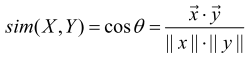高斯函数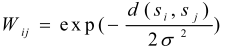图分割算法

常用的分割方法有：

最小分割法（Minimum Cut）

假如将一个图G 划分为A，B 两个子图，  那么目标函数可以理解为。此种方法对于规范的数据利用目标函数对图像进行分割效果较好，但对于非规范的数据此目标函数会出现偏向最小分割的结果。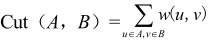规范化分割（Normalized Cut）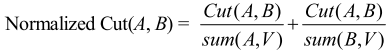其中Cut(A,B)表示 A，B 两个子图的相似程度，sumA 表示 A 图中所有点的权值之和，规范化分割方法对于非规范的数据也比较试用。

最小最大分割准则（Min-max Cut）

最小最大分割准则要求最小化Cut（A,B）的同时，最大化sum(A,A)与sum（B,B）。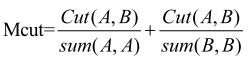比例分割法（Ratio Cut）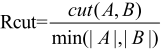其中|A|，|B|分别表示子图 A，B中顶点的个数，目标 Rcut  函数只简单考虑到如何使 A，B两个子图间的相似性最小，这样可以减少分割的次数。

谱聚类分割方法

谱聚类分割方法如下：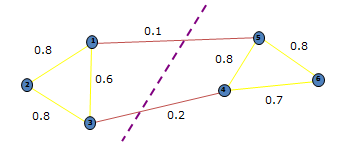（1）根据输入数据构建相似矩阵W；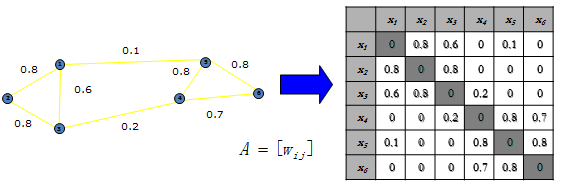（2）利用相似矩阵W 计算出对角矩阵D和规范化Laplacian 矩阵L； 关于Laplacian矩阵请参考图的Laplacian矩阵。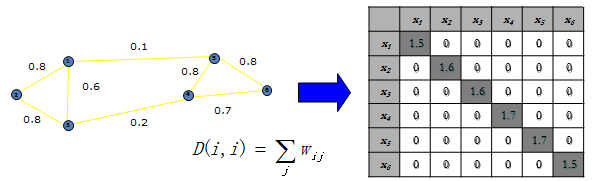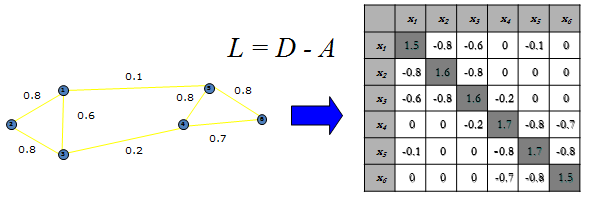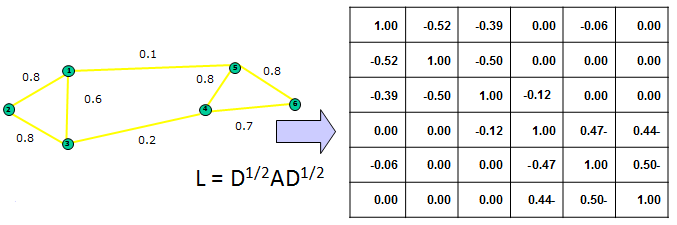（3）计算出矩阵L前K个最小特征值及其对应的特征向量，组成新的矩阵，矩阵的行数为样本数N，列数为K；

（4）利用Kmeans 算法进行聚类。

参考资料：
（1）A Tutorial on Spectral Clustering
（2）Learning Spectral Clustering
（3）Spectral Clustering
展开全文数据 数据分析
• ## Kmeans聚类算法详解

万次阅读 多人点赞 2018-05-16 18:41:40
摘要：本文通过图文详细介绍Kmeans聚类算法的原理和程序实现，以及如何选取类簇中心点。本文首先介绍利用该算法的原理及理解，详细介绍基于MATLAB设计一个自定义的Kmeans函数过程，然后利用该函数对UCI的数据集进行...人工智能
• 对主要聚类算法进行实现：基于划分的聚类算法，基于密度的聚类算法，基于层次的聚类算法。并且重点实现“基于快速搜索与寻找密度峰值的聚类”算法，并对其进行改进：自动获取聚类中心数
• 基于opencv3 实现 基于聚类的图像分割，k-means聚类效果好，但需要注意需认为设定聚类数量，可在代码中更改。
• 图聚类算法研究现状聚类分析是一种常用的机器学习技术，它的目的是将一个数据点划分为几个类。同一个类的数据之间具有较高的相似性，不同的类之间的相似度较低。很多研究已表明聚类是一种极具竞争力的聚类算法...
• 聚类算法建立在谱理论基础上，与传统的聚类算法相比，它具有能在任意形状的样本空间上聚类且收敛于全局最优解的优点。该算法首先根据给定的样本数据集定义一个描述成对数据点相似度的亲合矩阵,并且计算矩阵的...
• 以下是文章《基于密度峰值的混合型数据聚类算法设计》中的聚类效果的彩色，为方便感兴趣的读者查阅特别将其列出，谢谢！
• 文章目录图像聚类算法一、聚类二、聚类常见的算法2.1 K-Means聚类K-means聚类算法的分析流程：K-Means聚类与图像处理2.2 层次聚类2.3 密度聚类 图像聚类算法 一、聚类   从广义上说，聚类就是将数据集中某些方面...
• 点击上方“小白学视觉”，选择加"星标"或“置顶”重磅干货，第一时间送达 本文转自：磐创AI1. 典型聚类算法1.1 基于划分的方法代表：kmeans算法·指定k个聚类中心...人工智能 数据挖掘 机器学习
• 每篇一句： Time is always too short for those who need it, ... 近邻聚类法同样是一种基于距离阈值的聚类算法。问题：有N个待分类的模式{X1,X2,…,Xn}，要求按距离阈值T分类到以Z1,Z2,…为聚类中心的模式类中。
• ## 聚类算法总结

千次阅读 2020-12-27 02:28:20
文章目录聚类算法聚类算法简介认识聚类算法聚类算法的概念聚类算法与分类算法最大的区别聚类算法api初步使用api介绍案例聚类算法实现流程k-means聚类步骤案例练习小结模型评估误差平方和(SSE \The sum of squares ...python 机器学习
• 聚类算法(4)--Hierarchical clustering层次聚类 系统聚类：相当于自下而上法，也就是层次聚类 目录 一、系统聚类 1. 系统聚类实现的一般步骤 2. 常用的距离 3. 类间距离 二、手动实现过程 三、代码实现 1....机器学习 数据挖掘
• 聚类算法 文章目录聚类算法学习目标6.2 聚类算法api初步使用1 api介绍2 案例2.1流程分析2.2 代码实现 学习目标 掌握聚类算法实现过程 知道K-means算法原理 知道聚类算法中的评估模型 说明K-means的优缺点 了解聚类...机器学习 python 深度学习
• 聚类算法之——K-Means++聚类算法 轮盘法 基本思想：各个个体被选中的概率与其适应度大小成正比； 步骤 计算出群体中 每个个体的适应度f(i=1,2,…,m)，mf(i=1,2,\dots,m)，mf(i=1,2,…,m)，m为群体大小; 计算出...python 机器学习
• 聚类算法 文章目录聚类算法学习目标6.3 聚类算法实现流程1 k-means聚类步骤2 案例练习3 小结 学习目标 掌握聚类算法实现过程 知道K-means算法原理 知道聚类算法中的评估模型 说明K-means的优缺点 了解聚类中的算法...python 人工智能 机器学习
• 一、Kmeans聚类算法基本原理 K-Means算法的思想很简单，对于给定的样本集，按照样本之间的距离大小，将样本集划分为K个簇。让簇内的点尽量紧密的连在一起，而让簇间的距离尽量的大。 以彩色图像为例：基于彩色图像的...opencv c++
• 聚类算法总结学习sklearn给出的几种聚类算法的原理和特点学习资料： sklearn官方文档 affinity propagation Meanshift，聚类算法 meanshift算法图解以及在图像聚类、目标跟踪中的应用 K-means原理 把样本根据...机器学习
• 感谢博主 1.常见算法 ...此类算法假设聚类结构能够通过一组原型刻画，通常情形下，算法先对原型进行初始化，然后对原型进行迭代更新求解。–西瓜书 (1).K均值聚类（K-Means） 给定样本集D={x1,x2,..xn},...
• 这篇博客来一个汇总的实例，分别创建圆形数据、月牙形数据、聚团数据以及随机数据，并测试不同数据在各种不同聚类算法中的聚类效果以及消耗时间。 import time import warnings import numpy as np import ...
• 一、模糊C-means聚类算法 1.简介 模糊c-均值聚类算法 fuzzy c-means algorithm (FCMA)或称（ FCM）。在众多模糊聚类算法中，模糊C-均值（ FCM） 算法应用最广泛且较成功，它通过优化目标函数得到每个样本点对所有...
• 前言：Density Peaks聚类算法和DBSCAN聚类算法有相似的地方，两者都是基于密度的聚类方式。自己是在学习无监督学习过程中，无意间见到介绍这种聚类算法的文章，感觉Density Peaks聚类算法方法很新奇，操作也很简答，...无监督学习
• 我曾在机器学习之聚类算法应用篇中介绍过，聚类算法常使用轮廓系数来评估聚类效果，不过有时候并不是轮廓系数越大越好，如下面两幅所示，中的红色虚线表示聚类系数分数： 显然将簇数据设置为2的时候得到的...sklearn 可视化
• 聚类分析的算法可以分为划分法（Partitioning Methods）、层次法（Hierarchical Methods）、基于密度的方法（density-based methods）、基于网格的方法（grid-based methods）、基于模型的方法（Model-Based Methods...
• ## 各种聚类算法的介绍和比较

万次阅读 多人点赞 2017-11-15 15:25:00
一、简要介绍1、聚类概念聚类就是按照某个特定标准(如距离准则)把一个数据集分割成不同的类或簇，使得同一个簇内的数据对象的相似性尽可能大，同时不在同一个簇中的数据对象的差异性也尽可能地大。即聚类后同一类的......